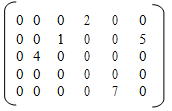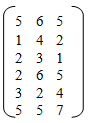## Representing sparse matrix in memory using array, Data Structure & Algorithms

Assignment Help:

Q. What do you understand by the term sparse matrix? How sparse matrix is stored in the memory of a computer? Write down the function to find out the transpose of a sparse matrix using this particular representation.

Ans.

Sparse Matrix is described below

A m x n matrix A would be a sparse if most of its elements are zero. A matrix that is not sparse is known as dense matrix.

Representing Sparse Matrix in Memory Using Array is described below

In an array representation an array of triplets of type < row, col, element> is used to store non-zero elements, where 1st field of the triplet is used to trace row position second to record column and the 3rd to record the non zero elements of  sparse matrix.

In addition, we are required to record the size of the matrix ( i.e. number of rows and the number of columns) and non zero elements of array of triplets are used for this purpose where the 1st filed saves the number of rows and the 2nd field saves the number of columns and the third field saves the number of non zero elements. The remaining elements of the array saves matrix on row major order. The array representation will be

[2 * (n+1) * size of (int) + n*size of(T)] bytes of memory where n is the number of non-zero elements and T is the data type of the element.

Ex: consider a 5*6 sparse matrix which is written belowArray Representation of Sparse Matrix is given belowHere n = 5 but the size of array is 6 as first row saves the order of array along with a number non-zero elements.

Memory declaration will be as followsas shown below

# define Max 50 struct triplet

{   int row;

int col;

float element;

}

struct triplet sparse_mat [MAX];

sparse matrix represented as above

[n is the number of non zero elements in array]

for I= 1,2,...n+1 temp = a[I].row a[I].row= a[I].col a[I].col = temp endfor.

#### A tree having ''m'' nodes has (m-1) branches. prove., Q. Prove the hypothes...

Q. Prove the hypothesis that "A tree having 'm' nodes has exactly (m-1) branches".      Ans: A tree having m number of nodes has exactly (m-1) branches Proof: A root

#### How will you represent a max-heap sequentially, How will you represent a ma...

How will you represent a max-heap sequentially? Max heap, also known as the descending heap, of size n is an almost complete binary tree of n nodes such that the content of eve

#### Applications in file systems of avl trees, 1. In computer science, a classi...

1. In computer science, a classic problem is how to dynamically store information so as to let for quick look up. This searching problem arises frequently in dictionaries, symbol t

#### Prims algorithm, how to implement prims algorithm dynamically

how to implement prims algorithm dynamically

#### Determination of time complexity, Determination of Time Complexity The...

Determination of Time Complexity The RAM Model The random access model (RAM) of computation was devised through John von Neumann to study algorithms. In computer science,

#### Darw a flowchart that inputs country someone is visiting, Regis lives in Br...

Regis lives in Brazil and frequently travels to USA, Japan and Europe. He wants to be able to convert Brazilian Reais into US dollars, European euros and Japanese yen. Conversion f

#### Space and time complexity, why the space increase in less time programs

why the space increase in less time programs

#### Push and pop operations, Q. Explain that how do we implement two stacks in ...

Q. Explain that how do we implement two stacks in one array A[1..n] in such a way that neither the stack overflows unless the total number of elements in both stacks together is n.

#### Define about the class invariant, Define about the class invariant A cl...

Define about the class invariant A class invariant may not be true during execution of a public operation though it should be true between executions of public operations. For

#### Multidimensional array, Q. The system allocates the memory for any of the m...

Q. The system allocates the memory for any of the multidimensional array from a big single dimensional array. Describe two mapping schemes that help us to store the two dimensi